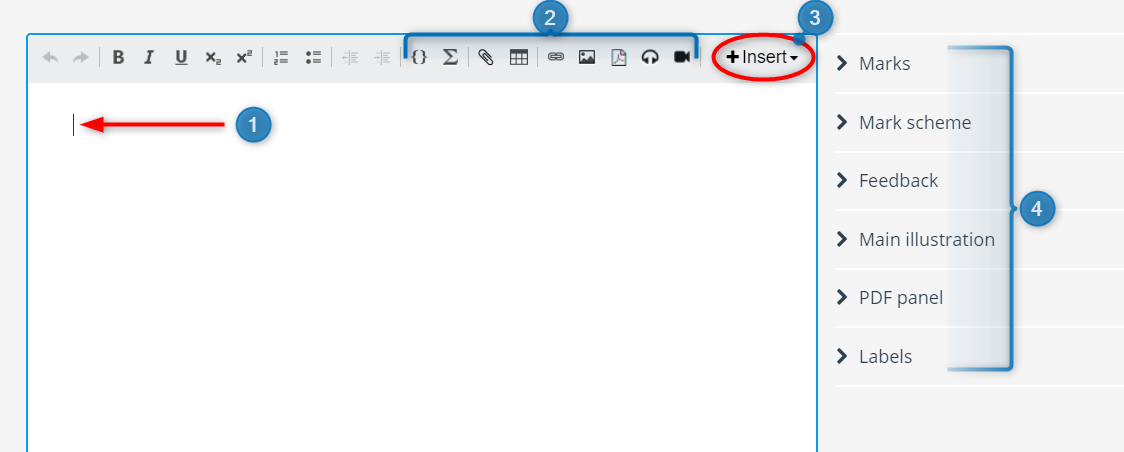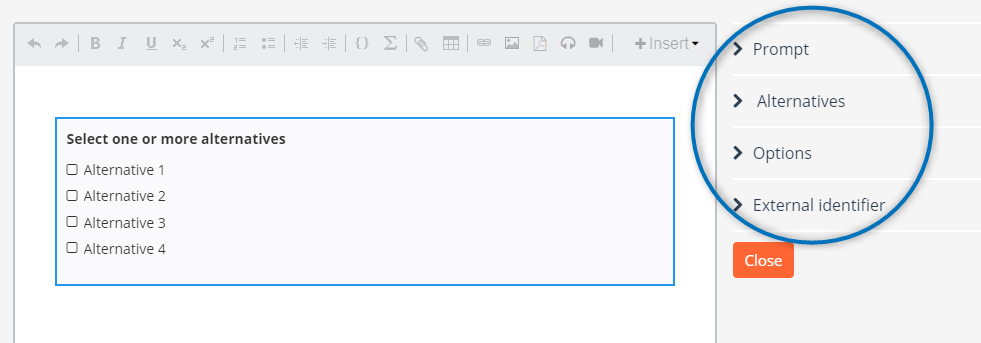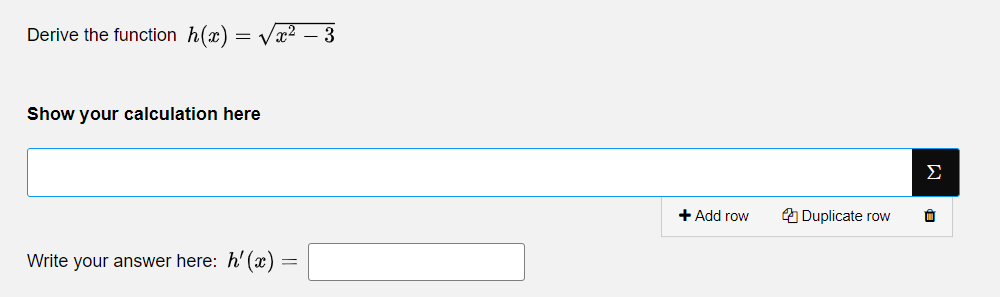# Question type - CompositeAutomatically marked question.  Question with the possibility to combine different question types.

Composite is a question type where several question types can be combined.

Composite is available for the following question types:

• Multiple Choice,
• Multiple Response
• True / false
• Text Entry
• Numeric Entry
• Math Entry
• Inline Choice
• Math Working

Combine different types and / or use several of one or more types.

In the question's edit mode, you can do the following:1. Write the desired question text.

2. Add math (LaTex), files, tables, links, PDF, images and / or audio clips to the question text.

3. Add one or more interaction elements (question types) by clicking +Insert.

Available question types are: Multiple Choice, Multiple Response, True / false, Text Entry, Numeric Entry, Math Entry, Inline Choice, and Math Working.

Note: Math Working is a manually marked question type and will not be automatically marked in a composite question. The purpose of including this question type is to have more flexibility for the candidates. Make sure you add at least one of the other question types. See Example of using the question type

4. Set general options for the question type. Read more about general options for questions

## Specific options

By clicking on the interaction element of the selected question type, you will get the specific options for the question type.In the example above, the question type Multiple Choice is inserted.

Specific options are about the same for each question type. Visit each question type to read more about their specific options:

Tip: Verify the automatic scoring for the question by answering the question in preview. The scoring cannot be changed after the test has started.

## Example of using the question type

The image below shows the candidate experience where the question types Math Entry and Math Working are composed.By combining these two question types, the candidates can show both the calculations and a written answer. This way, the answer to the question can be automatically marked while giving the grader the opportunity to see the candidates' calculations.

## Known limitations

The following limitations apply only to Multiple Choice and Multiple Response questions. In a Composite question, the two question types have different scoring logic.

This is best illustrated through an example:

If you create a composite question that has Marks per wrong answer set to -1, and Marks for unanswered set to 0, the following will occur:

• If the composite question is attempted and a Multiple Choice question is left unanswered, the scoring gives -1 per unanswered question element.
• If the composite question is attempted but a Multiple Response question is left unanswered, the scoring gives 0 per unanswered question element.